Want to share your content on R-bloggers? click here if you have a blog, or here if you don't.

K-Means Clustering is a well known technique based on unsupervised learning. As the name mentions, it forms ‘K’ clusters over the data using mean of the data. Unsupervised algorithms are a class of algorithms one should tread on carefully. Using the wrong algorithm will give completely botched up results and all the effort will go down the drain. Unlike supervised learning algorithms where one can still get around keeping some parts as an unknown black box, knowing the technique inside out starting from the assumptions made to the process, methods of optimization and uses is essential. So let us begin step by step starting from the assumptions. I will then explain the process and a hands-on illustration using R

## Assumptions and Process

Why do we assume in the first place? The answer is that making assumptions helps simplify problems and simplified problems can then be solved accurately. To divide your dataset into clusters, one must define the criteria of a cluster and those make the assumptions for the technique. K-Means clustering method considers two assumptions regarding the clusters – first that the clusters are spherical and second that the clusters are of similar size. Spherical assumption helps in separating the clusters when the algorithm works on the data and forms clusters. If this assumption is violated, the clusters formed may not be what one expects. On the other hand, assumption over the size of clusters helps in deciding the boundaries of the cluster. This assumption helps in calculating the number of data points each cluster should have. This assumption also gives an advantage. Clusters in K-means are defined by taking the mean of all the data points in the cluster. With this assumption, one can start with the centers of clusters anywhere. Keeping the starting points of the clusters anywhere will still make the algorithm converge with the same final clusters as keeping the centers as far apart as possible.

Now let’s understand how the algorithm works. The first step is to assign initial clusters. You can specify any K clusters or let the algorithm assign them randomly. The algorithm works in iterations and in every iteration, all the data points are then assigned to one of the clusters based on the nearest distance from the centers. After all points are assigned to one of the cluster, the cluster centers are now updated. The new centers are decided based on the centroid mean of all the points within the cluster. This is repeated, iteration after iteration until the there is no change in the cluster assignment of any of the data points but there are a lot of calculations which are not fixed in this algorithm. For example, one can decide how the distance for each data point from the cluster center is defined. All of us are familiar with the Euclidean distance. The algorithm is straightforward and easy to understand but using the technique is not as easy as it looks. Let’s try out some examples in R.

K-Means Starter
To understand how K-Means works, we start with an example where all our assumptions hold. R includes a dataset about waiting time between eruptions and the duration of the eruption for the Old Faithful geyser in Yellowstone National Park known as ‘faithful’. The dataset consists of 272 observations of 2 features.
```#Viewing the Faithful dataset
plot(faithful)
```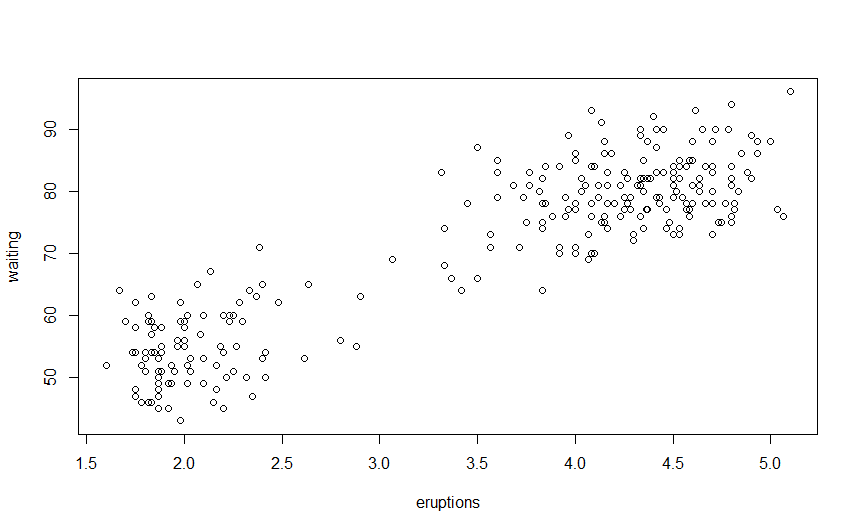Looking at the dataset, we can notice two clusters. I will now use the kmeans() function in R to form clusters. Let’s see how K-Means clustering works on the data
```#Specify 2 centers
k_clust_start=kmeans(faithful, centers=2)
#Plot the data using clusters
plot(faithful, col=k_clust_start\$cluster,pch=2)```Being a small dataset, clusters are formed almost instantaneously but how do we see the clusters, their centers or sizes? The k_clust_start variable I used contains information on both centers and the size of clusters. Let’s check them out
```#Use the centers to find the cluster centers
k_clust_start\$centers

eruptions      waiting
1       4.29793     80.28488
2       2.09433     54.75000

#Use the size to find the cluster sizes
k_clust_start\$size
 172 100
```
This means the first cluster consists of 172 members and is centered at 4.29793 value of eruptions and 80.28488 value of waiting. Similarly the second cluster consists of 100 members with 2.09433 value of eruptions and 54.75 value of waiting. Now this information is golden! We know that these centers are the cluster means. So, the eruptions typically happen for either ~2 mins or ~4.3 mins. For longer eruptions, the waiting time is also longer.

## Getting into the depths

Imagine a dataset which has clusters which one can clearly identify but k-means cannot. I’m talking about a dataset which does not satisfy the assumptions. A common example is a dataset which represents two concentric circles. Let’s generate it and see how it looks like
```#The following code will generate different plots for you but they will be similar
library(plyr)
library(dplyr)
#Generate random data which will be first cluster
clust1 = data_frame(x = rnorm(200), y = rnorm(200))
#Generate the second cluster which will ‘surround’ the first cluster
clust2 =data_frame(r = rnorm(200, 15, .5), theta = runif(200, 0, 2 * pi),
x = r * cos(theta), y = r * sin(theta)) %>%
dplyr::select(x, y)
#Combine the data
dataset_cir= rbind(clust1, clust2)
#see the plot
plot(dataset_cir)
```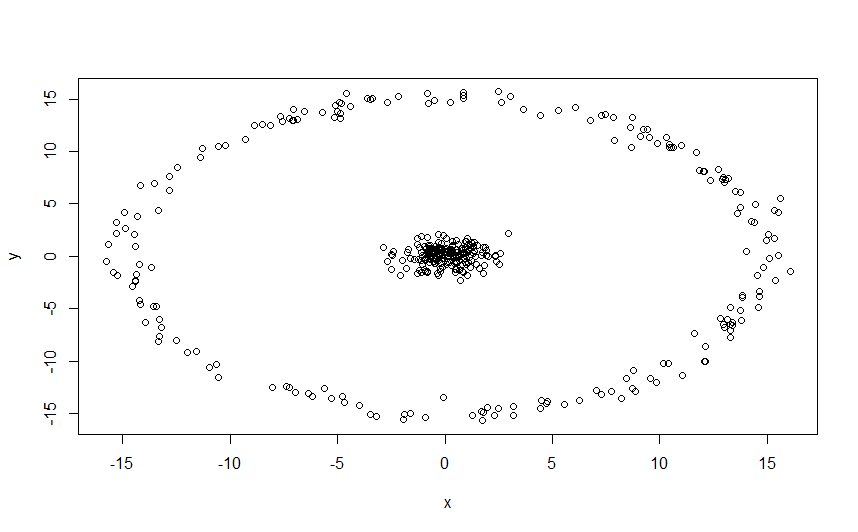Simple, isn’t it? There are two clusters – one in the middle and the other circling the first. However, this violates the assumption that the clusters are spherical. The inner data is spherical while the outer circle is not. Even though the clustering will not be good, let’s see how does k-means perform on this data
```#Fit the k-means model
k_clust_spher1=kmeans(dataset_cir, centers=2)
#Plot the data and clusters
plot(dataset_cir, col=k_clust_spher1\$cluster,pch=2)
```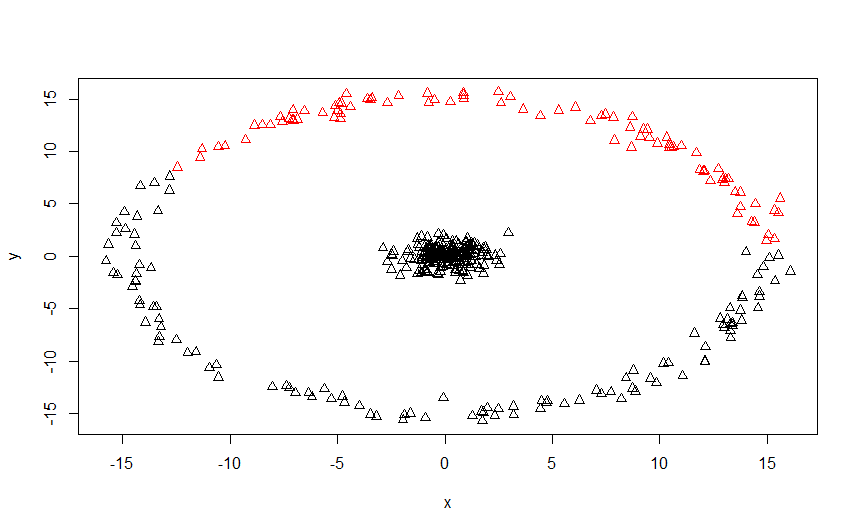How do we solve this problem? There are clearly 2 clusters but k-means is not working well. A simple way in this case is to transform our data into polar format. Let’s convert it and plot it.
```#Using a function for transformation
cart2pol=function(x,y){
#This is r
newx=sqrt(x^2 + y^2)
#This is theta
newy=atan(y/x)
x_y=cbind(newx,newy)
return(x_y)
}
dataset_cir2=cart2pol(dataset_cir\$x,dataset_cir\$y)
plot(dataset_cir2)
```Now we run the k-means model on this data
```k_clust_spher2=kmeans(dataset_cir2, centers=2)
#Plot the data and clusters
plot(dataset_cir2, col=k_clust_spher2\$cluster,pch=2)
```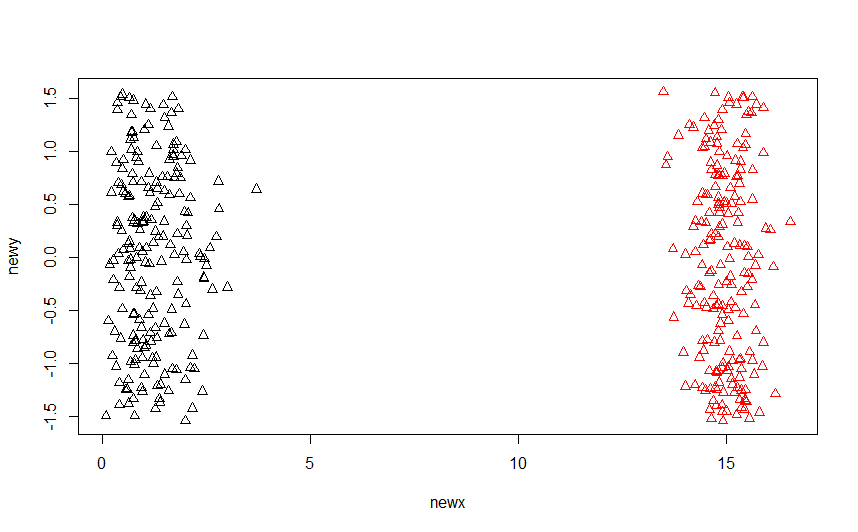This time k-means algorithm works well and correctly transform the data. We can also view the clusters on the original data to double-check this.
```plot(dataset_cir, col=k_clust_spher2\$cluster,pch=2)
```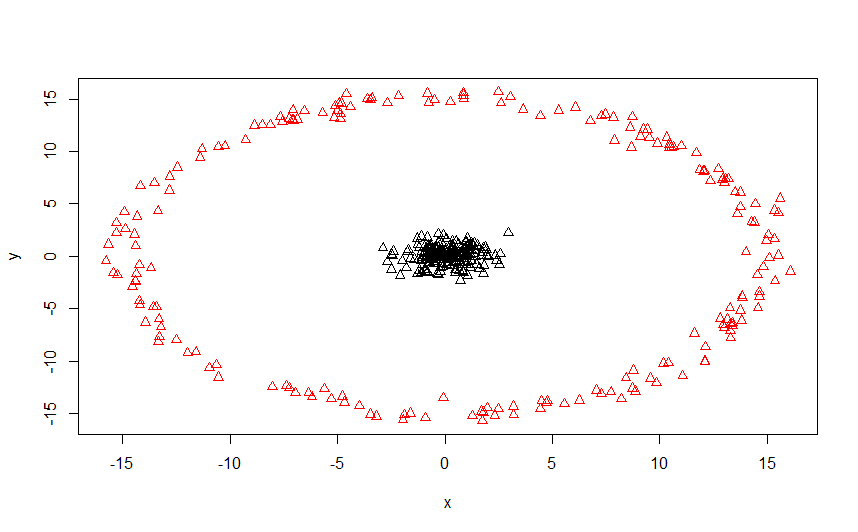By transforming our data into polar coordinates and fitting k-means model on the transformed data, we fulfil the spherical data assumption and data is accurately clustered. Now let’s look at a data where the clusters are not of similar sizes. Similar size does not mean that the clusters have to be exactly equal. It simply means that no cluster should have ‘too few’ members.
```#Make the first cluster with 1000 random values
clust1 = data_frame(x = rnorm(1000), y = rnorm(1000))
#Keep 10 values together to make the second cluster
clust2=data_frame(x=c(5,5.1,5.2,5.3,5.4,5,5.1,5.2,5.3,5.4),y=c(5,5,5,5,5,4.9,4.9,4.9,4.9,4.9))
#Combine the data
dataset_uneven=rbind(clust1,clust2)
plot(dataset_uneven)
```Here again, we have two clear clusters but they do not satisfy similar size requirement of k-means algorithm.
```k_clust_spher3=kmeans(dataset_uneven, centers=2)
plot(dataset_uneven, col=k_clust_spher3\$cluster,pch=2)
```Why did this happen? K-means tries to minimize inter cluster and intracluster distance and create ‘tight’ clusters. In this process, it assigns some data points in the first cluster to the second cluster incorrectly. This makes the clustering inaccurate.

## How to decide the value of K in data

The datasets I worked on in this article are all simple and it is easily to identify clusters by plotting them. However, complicated datasets do not have this luxury. The Elbow method is popular for finding a suitable value of ‘K’ for k-means clustering. This method uses SSE within groups for different values of k and plots them. Using this plot, we can choose the ‘k’ which shows an abrupt change in SSE, creating an ‘elbow effect’. I will show an illustration on iris dataset using petal width and petal length.
```#Create a vector for storing the sse
sse=vector('numeric')
for(i in 2:15){
#k-means function in R has a feature withinss which stores sse for each cluster group
sse[i-1]=sum(kmeans(iris[,3:4],centers=i)\$withinss)
}
#Converting the sse to a data frame and storing corresponding value of k
sse=as.data.frame(sse)
sse\$k=seq.int(2,15)
#Making the plot. This plot is also known as screeplot
plot(sse\$k,sse\$sse,type="b")
```In this plot, the first elbow is formed at k=3. This method suggest that we should have 3 clusters for this data.

## Conclusion

K-means clustering is one of the first and most basic clustering techniques whenever one thinks of unsupervised clustering. However, this technique is not just powerful, but also teaches the importance of understanding the data in unsupervised learning. If any of the assumptions are violated then the clusters are not formed properly. Similarly, while determining the value of ‘k’, using improper or arbitrary values may lead to improper clusters. In a way, k-means clustering also relies on the correct value of k for clustering the data accurately. Unsupervised learning is fun but not a wild attempt. One must be clear of all aspects of the algorithm and its assumptions before implementing it rather than treating it as a black box and shooting in the dark. This article illustrates the various shortcomings of improperly clustering data on simple datasets which do not satisfy the assumptions of the algorithm. The full code used in this article is given below.
```#Viewing the Faithful dataset
plot(faithful)

#Specify 2 centers
k_clust_start=kmeans(faithful, centers=2)
#Plot the data using clusters
plot(faithful, col=k_clust_start\$cluster,pch=2)

#Use the centers to find the cluster centers
k_clust_start\$centers
#Use the size to find the cluster sizes
k_clust_start\$size
#The following code will generate different plots for you but they will be similar

library(plyr)
library(dplyr)
#Generate random data which will be first cluster
clust1 = data_frame(x = rnorm(200), y = rnorm(200))
#Generate the second cluster which will ‘surround’ the first cluster
clust2 =data_frame(r = rnorm(200, 15, .5), theta = runif(200, 0, 2 * pi),
x = r * cos(theta), y = r * sin(theta)) %>%
dplyr::select(x, y)
#Combine the data
dataset_cir= rbind(clust1, clust2)
#see the plot
plot(dataset_cir)

#Fit the k-means model
k_clust_spher1=kmeans(dataset_cir, centers=2)
#Plot the data and clusters
plot(dataset_cir, col=k_clust_spher1\$cluster,pch=2)

#Using a function for transformation
cart2pol=function(x,y){
#This is r
newx=sqrt(x^2 + y^2)
#This is theta
newy=atan(y/x)
x_y=cbind(newx,newy)
return(x_y)
}
dataset_cir2=cart2pol(dataset_cir\$x,dataset_cir\$y)
plot(dataset_cir2)

k_clust_spher2=kmeans(dataset_cir2, centers=2)
#Plot the data and clusters
plot(dataset_cir2, col=k_clust_spher2\$cluster,pch=2)

plot(dataset_cir, col=k_clust_spher2\$cluster,pch=2)

#Make the first cluster with 1000 random values
clust1 = data_frame(x = rnorm(1000), y = rnorm(1000))
#Keep 10 values together to make the second cluster
clust2=data_frame(x=c(5,5.1,5.2,5.3,5.4,5,5.1,5.2,5.3,5.4),y=c(5,5,5,5,5,4.9,4.9,4.9,4.9,4.9))
#Combine the data
dataset_uneven=rbind(clust1,clust2)
plot(dataset_uneven)

k_clust_spher3=kmeans(dataset_uneven, centers=2)
plot(dataset_uneven, col=k_clust_spher3\$cluster,pch=2)

#Create a vector for storing the sse
sse=vector('numeric')
for(i in 2:15){
#k-means function in R has a feature withinss which stores sse for each cluster group
sse[i-1]=sum(kmeans(iris[,3:4],centers=i)\$withinss)
}
#Converting the sse to a data frame and storing corresponding value of k
sse=as.data.frame(sse)
sse\$k=seq.int(2,15)
#Making the plot. This plot is also known as scree-plot
plot(sse\$k,sse\$sse,type="b")
```